# C. Details of the Model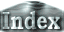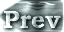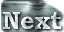### f. Upper Damping Layers

In order to thoroughly eliminate noise in the upper layers, Rayleigh friction and Newtonian cooling type smoothing are included in the top seven layers. Specifically, the terms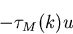and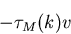are added to the x- and y-components of the equations of motion, respectively, and the term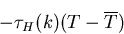is added to the equation of temperature. Here,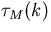and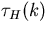represent the relaxation time for damping and smoothing, respectively, and are functions of height.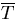represents zonal mean temperature. The values ofandin Table 1 are used for calculations. The values ofandadopted for the upper-most layer, which is 3 hours, are based on the SKYHI general circulation model of Geophysical Fluid Dynamics Laboratory (Manzini and Hamilton, 1993). Furthermore, the choice of the number of layers into which the Rayleigh friction and Newtonian cooling type smoothing are incorporated is based on the results from a number of test experiments. If the upper damping layer consists of only five layers, integration over a long time period becomes infeasible for the case of S = 1800 W/m2.
verical level τm, τH
k = 32   10800
k = 31   21600
k = 30   32400
k = 29   43200
k = 28   54000
k = 27   64800
k = 26   75600
Table 1: Relaxation time for the upper damping layer. k = 32 indicates the upper-most layer. The relaxation time for this layer is 3 hours.

 C.f. Upper Damping Layers